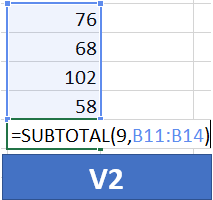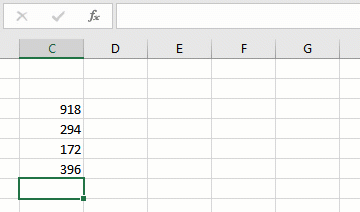# SUBTOTAL Macro v2The SUBTOTAL function is one of my favorites. So, I wrote a post about it a while back that provided a macro to insert it. The macro worked kinda like the AutoSum command, but it inserted the SUBTOTAL function instead of the SUM function. Gary posted a suggestion for enhancing the macro, so, I wrote this follow up post.

In summary, Gary asked for a tweak to the original macro to enable him to manually select the sum range. So, this version of the macro does just that … it will insert the SUBTOTAL function into the active cell, but, prompt you to select the cells you want to sum. Thanks Gary … and let’s jump into the VBA code 🙂

Note: If you haven’t seen the original post, it is here just in case you’d like to check it out for reference or a quick background.

## Macro

Before we jump right to the code, let’s see what the macro does. The macro writes the SUBTOTAL function into the active cell after prompting the user to select the sum range, as shown below:There are actually only a few lines of code needed to make this macro work. So, I’ll walk through them and explain what they do. But, as with most macros, there is additional code required if you want to handle exceptions and errors, declare variables, provide comments, and make it more user-friendly. So, if you are interested in the enhanced version of the macro, just download the sample file at the end of the post.

Let’s start by looking at the basic macro, and then we’ll walk through each line:

```
Sub SubtotalSelect()
Set SumRange = Application.InputBox("Select sum range", Type:=8)
ActiveCell.Formula = "=SUBTOTAL(9," & SumRange.Address & ")"
End Sub
```

The vba code basically does three things:

• It defines the macro name
• It asks the user for the sum range
• It writes the formula into the active cell

Let’s take them one by one.

### Define the macro name

The first and last lines, Sub SubtotalSelect() and End Sub, provide the macro name SubtotalSelect and enclose the related VBA code.

### Ask user for sum range

The next line has two parts. The first part, Set SumRange, is telling Excel that we want to store something in a variable named SumRange. It is best practice to actually declare this variable before using it, which is done in the enhanced version available for download below. We’ll use the SumRange variable to store the user-selected sum range.

The next part Application.InputBox(“Select sum range”, Type:=8) tells Excel to display a small dialog box which allows the user to select a range with the mouse. The first argument of the InputBox function is the message to display to the user, so, we can use a friendly message like “Select sum range” or similar. The Type:=8 argument tells Excel that we are expecting a range to be provided by the user, as opposed to a number or text string, etc.

The user’s selection is stored in the SumRange variable, which we’ll use to insert the formula into the active cell.

### Write formula into active cell

The next line writes the formula into the active cell and has two parts. The first part, ActiveCell.Formula tells Excel that we are about to provide the formula for the active cell.

The next part, “=SUBTOTAL(9,” & SumRange.Address & “)”, provides the formula. If you look carefully, you’ll see the formula is actually made up of 3 components combined with the concatenation operator (&), as follows:

1. “=SUBTOTAL(9,”
3. “)”

The first text string, “=SUBTOTAL(9,” tells Excel to insert the SUBTOTAL function, and to use 9 as the function’s first argument. 9 tells the function we want to sum the values in the range.

The second value is SumRange.Address, which is the address of the range selected by the user in the dialog.

The third value “)” simply closes the SUBTOTAL function.

And, that’s the macro!

Now, in addition to the basic macro displayed above, I’ve written an enhanced version which is available for download below that incorporates some error handling, variable declaration, and also tries to guess the default range by looking in the cells above the active cell. Here’s how it looks when you run it (notice the default range is pre-populated with the cells above):## Using the Macro

To use this macro, you’ll want to paste the code into your personal macro workbook or a macro toolbox, both described in a previous post here.

Plus, to make the macro easy to run, you can set up a QAT button. If you’d like to explore that, the steps are provided at the bottom of this post.

If you have any other suggestions or improvements, please share by posting a comment below.

## Sample Files

Notes:

• Each sample file contains two macros, the SubtotalSelect as discussed above, plus, the SubtotalSelectEnhanced which provides some error handling and other stuff.
• The Normal Excel file should be able to be downloaded and opened on most computers and networks, but, the macro won’t “run” until you paste the code into a vba module.
• The Macro-enabled Excel file may be blocked on some networks/computers, but, if you are able to download it and open it then the macro should run when you click the corresponding worksheet button.

Get a quick email notice when a new Excel article is available

• This field is for validation purposes and should be left unchanged.1.sandeep kothari